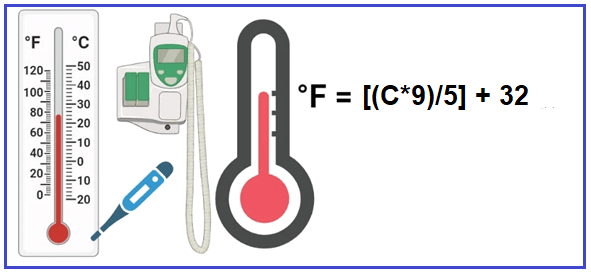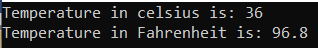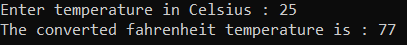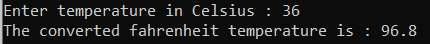# Celsius to Fahrenheit in C#

## Celsius to Fahrenheit in C# with Examples

In this article, I am going to discuss how to convert Celsius to Fahrenheit in C# with Examples. Please read our previous article, where we discussed How to Calculate the Volume of Cone in C#.

##### How to Convert Celsius to Fahrenheit in C#?

Celsius scale is a temperature scale based on the freezing point of water at 0 degrees centigrade and the boiling point of water at 100-degree centigrade. Fahrenheit scale is a temperature scale that is based on the freezing point of water at 32-degree Fahrenheit and the boiling point of water at 212-degree Fahrenheit.

The formula of Celsius to Fahrenheit is F = [(C*9)/5] + 32, Where F is degree Fahrenheit and C is Celsius Fahrenheit. For better understanding, please have a look at the below image.##### Example: Celsius to Fahrenheit

The formula of Celsius to Fahrenheit is: F = [(C*9)/5] + 32 = 96.8

In this program, we can use the following data type

1. Float: Floating-point numbers are used for decimal and exponential values. It is a primitive data type. It has 6 decimal digits of precision. Example: float volume = 20.93333
2. Double: Double is also a data type that is used to represent the floating-point numbers. It has 15 decimal digits of precision. Example: double y = 20.9333333333333
##### Logic to convert Celsius to Fahrenheit

We need to follow the below steps

1. We will define Celsius and Fahrenheit variables.
2. Then we will assign the value of Celsius.
3. Then we will calculate Fahrenheit by using the formula: F = [(C*9)/5] + 32
4. And the resulting output will store in the Fahrenheit variable.
##### Example:

The following C# Program will Convert Celsius to Fahrenheit

```using System;
class CelsiusToFahrenheit
{
static void Main()
{
float celsius = 36;
Console.WriteLine("Temperature in celsius is: " + celsius);
float fahrenheit = ((celsius * 9) / 5) + 32;
Console.WriteLine("Temperature in Fahrenheit is: " + fahrenheit);
}
}
```
###### Output:##### Example: Taking input from the user

In the following C# program, we will take the Celsius from the user and then calculate the Fahrenheit and print it on the console.

```using System;
namespace Celsius
{
class CelsiusToFahrenheit
{
static void Main(string[] args)
{
Console.Write("Enter temperature in Celsius : ");
double fahrenheit = ((celsius * 9) / 5) + 32;
Console.WriteLine("The converted fahrenheit temperature is : " + fahrenheit);
}
}
}
```

Output:##### C# Code (Using function)

In the below example, we have created one method to calculate Fahrenheit from Celsius.

```using System;
namespace Celsius
{
class CelsiusToFahrenheit
{
static void Main(string[] args)
{
Console.Write("Enter temperature in Celsius : ");
double fahrenheit = CelsiusFahrenheit(celsius);
Console.WriteLine("The converted fahrenheit temperature is : " + fahrenheit);
}

private static double CelsiusFahrenheit(double celsius)
{
return ((celsius * 9) / 5) + 32;
}
}
}
```
###### Output:In the next article, I am going to discuss How to Convert Fahrenheit to Celsius in C# with Examples. Here, in this article, I try to explain how to convert Celsius to Fahrenheit in C# with Examples and I hope you enjoy this article.